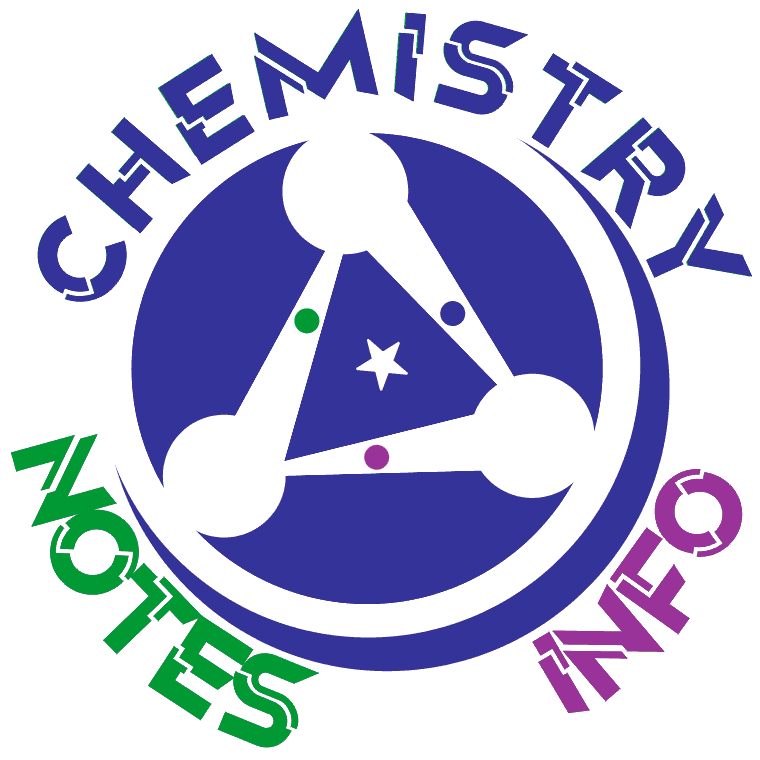NOTE:

If error occur=> Change website url from chemistrynotesinfo.blogspot.in to

Chemistry General Knowledge

Chemistry GK by www.ChemistryNotesInfo.com
This chemistry GK part8 is based on different measuring devices or measuring instruments used in chemistry and science.

Q1.Structure of crystals is measured by

• Crystal Gauge
• Diffractometer
• Diffractoscale
• Dynamometer
Ans of Chemistry GK MCQ Question Q1: Diffractometer

Q2.Size, speed, and velocity of raindrops is measured by

• Rainmeter
• Watermeter
• Rain Gauge
• Disdrometer
Ans of Chemistry GK MCQ Question Q2: Disdrometer

Q3.Volume changes caused by any physical or chemical process is measured by

• Dilatometer
• Caliper
• Densimeter
• Volume Sensor
Ans of Chemistry GK MCQ Question Q3: Dilatometer

Q4. ______ is used in measurement of rate of evaporation

• Barometer
• Heatingmeter
• Evaporationmeter
• Evaporimeter
Ans of Chemistry GK MCQ Question Q4: Evaporimeter

Q5.Exposure to hazards, especially radiation is measured by

• Polometer
• Bolometer
• Dosimeter
Ans of Chemistry GK MCQ Question Q5: DosimeterQ6.____ device is used to measure force,torque or power

• Dynamometer
• Forcealyzer
• Deltameter
• Fathometer
Ans of Chemistry GK MCQ Question Q6: Dynamometer

Q7. _______ is use to measure specific gravity of oils.

• Actinometer
• Elaeometer
• Specificgravity Analyser
• Densimeter
Ans of Chemistry GK MCQ Question Q7: Elaeometer

Q8. Device use to measure pitch of musical notes is

• Electronicmeter
• Electronic tuner
• DJ
• Buffer
Ans of Chemistry GK MCQ Question Q8: Electronic tuner

Q9. ______ is use to measure change in volume of gas mixture following combustion

• Gasometer
• Eudiometer
• Electronicmeter
• Electronic tuner
Ans of Chemistry GK MCQ Question Q9: Eudiometer

Q10.Refractive index, dielectric function and thickness of thin films is measured by

• Refractometer
• Ellipsometer
• Densimeter
• Dielectrometer
Ans of Chemistry GK MCQ Question Q10: Ellipsometer

We publish some chemistry general knowledge question-answer (Chemistry MCQs) to increase your chemical science GK.